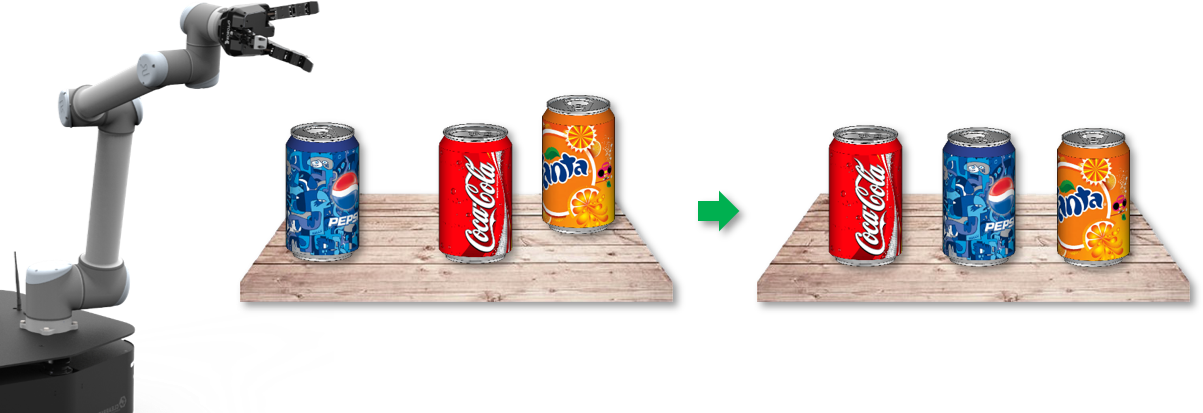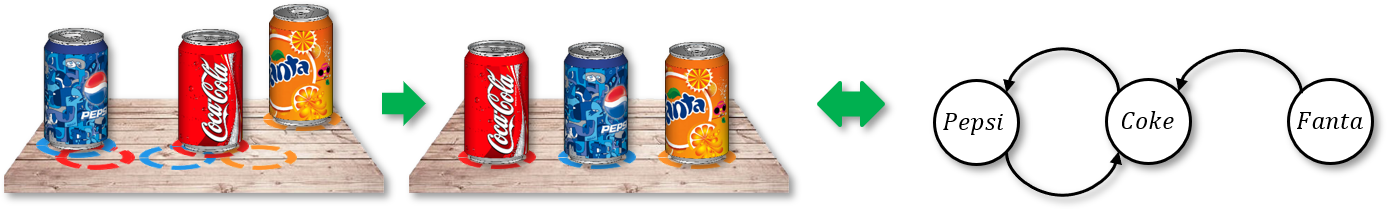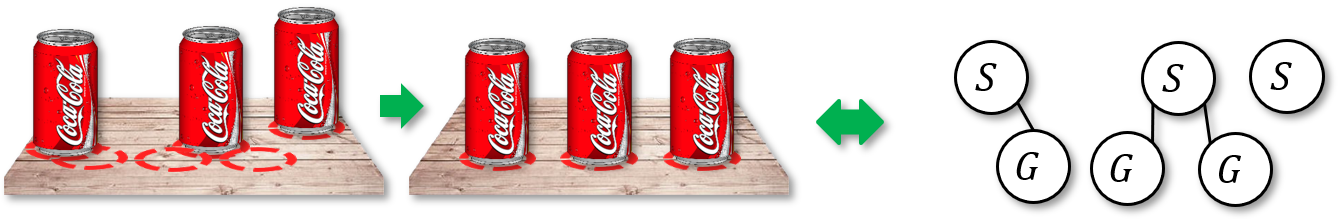# 余京津 | Jingjin Yu

View the Project on GitHub

## On Minimizing the Number of Running Buffers for Tabletop Rearrangement

In nearly all aspects of our everyday lives, be it work related, at home, or for play, objects are to be grasped and rearranged, e.g., tidying up a messy desk, cleaning the table after dinner, or solving a jigsaw puzzle. Similarly, many industrial and logistics applications require repetitive rearrangements of many objects, e.g., the sorting and packaging of products on conveyors with robots, and doing so efficiently is of critical importance to boost the competitiveness of the stakeholders. However, even without the challenge of grasping, deciding the sequence of objects for optimally solving a pick-n-place based rearrangement task is non-trivial.

For the example below, perhaps we want to arrange the sodas from the configuraiton on the left to the neater configuration on the right (the order between coke and pepsi does not necessarily reflect my personal preference :D). Let us assume the robot will use overhand grasps: it will pick up an object, lift it above all other objects, move it around, and drop it off at a place on the table that is collision-free. A natural question to ask here is, how many such pick-n-place operations are needed to solve a given problem?In solving the problem, we first make the following observation: the coke occupies the pepsi’s goal and vice versa, which means that one of them must be moved to a temporary location before the problem can be solved. This creates a two-way constraint. The coke also occupies the goal of the fanta, but this constraint is a one-way constraint. From the observation, we may fully capture the constraints in a tabletop pick-n-place rearrangement problem using dependency graphs, as shown on the right side of the figure below. Dependency graphs can be computed easily by extracting the the pairwise object constraints.The above dependency graph structure is induced by labeled (distinguishable) objects. When the objects are unlabeled, dependency graphs can also be defined. In this case, the dependency graphs are undirected and bipartite.As it turns out, minimizing the total number of objects to place at temporary locations, or buffers, is a difficult problem to solve at scale, because it is essentially the same as the feedback vertex set problem on the corresponding dependency graph, which is one of the first batch of problems proven to be NP-hard. This aspect of the tabletop rearrangement problem is studied by Han et al. a few years back.

Here, we are interested in a related optimization objective. Instead of the total number of buffers, what about the minimum number of buffers that are currently being used? That is, how many running buffers are needed to solve a given tabletop rearrangement problem? This is an important question to ask, because the number of running buffers is more relevant than the number of total buffers in deciding the feasibility of solving a given problem. That is, even if the total number of buffers may be very large, it is likely that at any moment, only a few objects needs to be displaced to solve the problem.

In a recent work,

On Minimizing the Number of Running Buffers for Tabletop Rearrangement
Kai Gao, Siwei Feng, Jingjin Yu. R:SS 2021.


we made a first systmatic study of the problem of minimizing the number of running buffers in solving pick-n-place based tabletop rearrangement (TORO) problem. As a summary of the results, we begin by showing that computing an MRB (minimum running buffer) solution on arbitrary dependency graphs is NP-hard. Then, we establish that for an $n$-object TORO instance, MRB can be lower bounded by $\Omega(\sqrt{n})$ for uniform cylinders, even when all objects are unlabeled. This implies that the same is true for the labeled setting. We also provide a matching algorithmic upper bound $O(\sqrt{n})$ for the unlabeled setting. In terms of practical methods, we have developed multiple highly effective and optimal algorithms for computing MRB rearrangement plans. In particular, we present a depth-first-search dynamic programming routine that readily scales to instances with over a hundred objects for the labeled setting, and a priority queue-based modification of a dynamic programming algorithm for the unlabeled setting. This allows us to quickly compute high-quality plans for solving highly constrained rearrangement problems like the follwoing, where only two external buffers are needed.